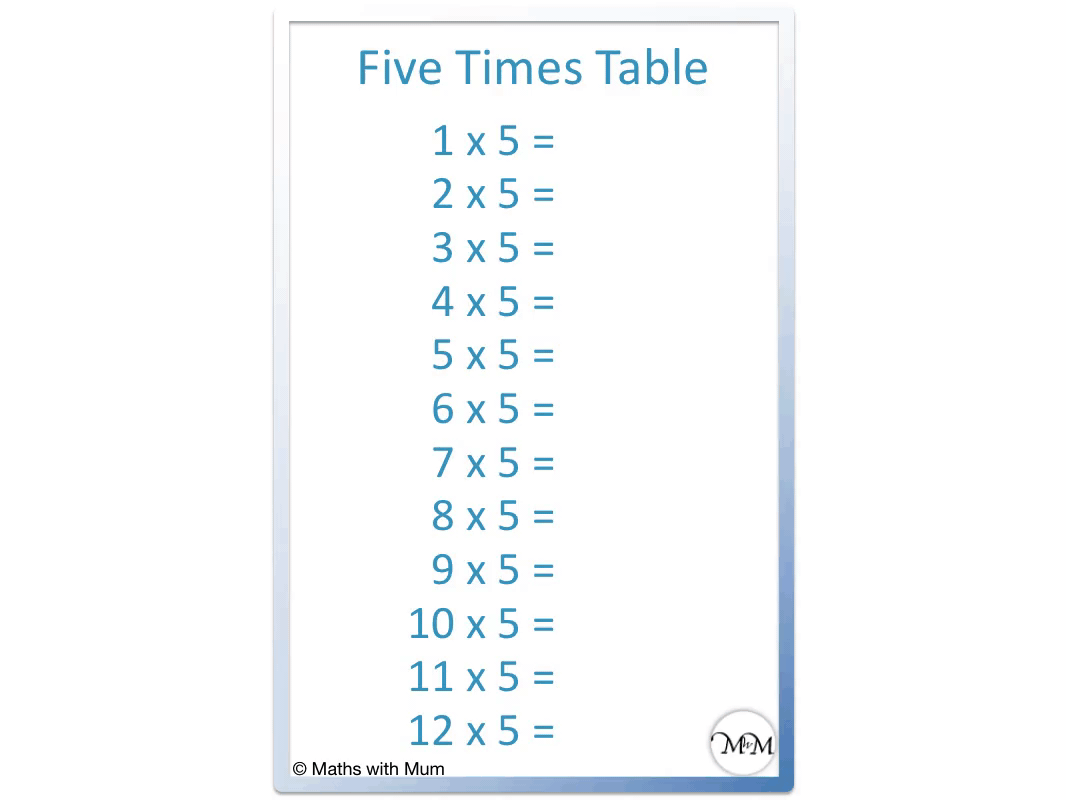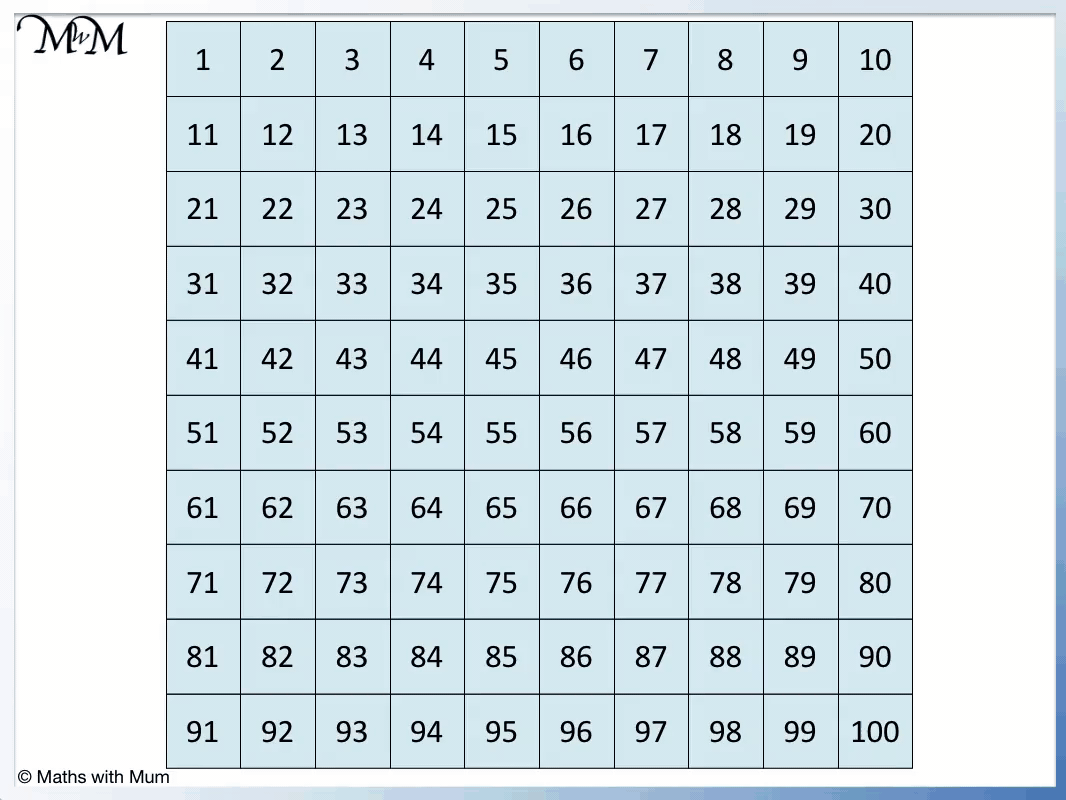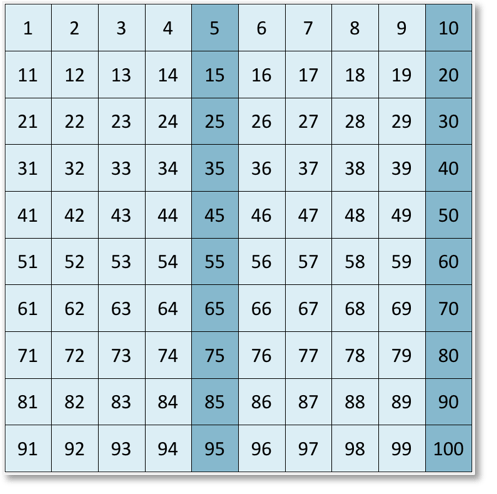# Multiples of 5

Multiples of 5• The multiples of 5 are numbers that can be divided exactly by 5, leaving no remainder.
• The first few multiples of 5 are the numbers in the 5 times table.
• For example 2 × 5 = 10 and so, the second multiple of 5 is 10.
• For example 100 × 5 = 500 and so, the hundredth multiple of 5 is 500.
• The multiples of 5 always end in 0 or 5.

A multiple of 5 is any number that can be divided by 5 exactly, leaving no remainder.#### Multiples of 5 Flashcards

Click on the multiples of 5 flashcards below to memorise the 5 times table:

×
×

=#### Multiples of 5: Interactive Questions

Multiples of 5: Interactive Questions

# Multiples of 5

## What are Multiples of 5?

Multiples of 5 are numbers that can be divided by 5 exactly, leaving no remainder. They are made by multiplying any whole number by 5. For example, the third multiple of 5 is 15 because 3 × 5 = 15. The multiples of 5 always end in 0 or 5.

The first few multiples of 5 are simply the 5 times table.The first multiples of 5 are:

• 1 × 5 = 5
• 2 × 5 = 10
• 3 × 5 = 15
• 4 × 5 = 20
• 5 × 5 = 25
• 6 × 5 = 30
• 7 × 5 = 35
• 8 × 5 = 40
• 9 × 5 = 45
• 10 × 5 = 50
• 11 × 5 = 55
• 12 × 5 = 60## Rule to Test if a Number is a Multiple of 5

Multiples of 5 only end in 5 or 0. If a number does not end in 5 or 0, it is not a multiple of 5. For example, 3470 is a multiple of 5 because it ends in a 0. The number 5526 is not a multiple of 5 because it ends in a 6.

Multiples of 10 are always multiples of 5. This is because 5 divides exactly into 10.

## How to Find Multiples of 5

To find multiples of 5, multiply any whole number by 5. For example, the tenth multiple of 5 is 50 because 10 × 5 = 50. Alternatively, the multiples of 5 can be found by starting from 0 and counting up in fives.

Here we have the first multiples of 5 up to 100 shown on a chart.

We start at 0 and add on 5 each time to get from one multiple to the next.

0 + 5 = 5. Then 5 + 5 = 10. Then 10 + 5 = 15 and so on.When teaching the multiples of 5, a number grid can be useful because we can easily see patterns in the position of the numbers. This helps us identify the multiples of 5. We can also see clearly that the multiples of 5 always end in a 5 or a 0.## What are the Multiples of 5 up to 100?

There are 20 multiples of 5 up to 100, which are:

5, 10, 15, 20, 25, 30, 35, 40, 45, 50, 55, 60, 65, 70, 75, 80, 85, 90, 95 and 100.

We can see that there is a difference of 5 between each multiple of 5.

Here is a longer list of the first 100 multiples of 5:

5, 10, 15, 20, 25, 30, 35, 40, 45, 50, 55, 60, 65, 70, 75, 80, 85, 90, 95, 100, 105, 110, 115, 120, 125, 130, 135, 140, 145, 150, 155, 160, 165, 170, 175, 180, 185, 190, 195, 200, 205, 210, 215, 220, 225, 230, 235, 240, 245, 250, 255, 260, 265, 270, 275, 280, 285, 290, 295, 300, 305, 310, 315, 320, 325, 330, 335, 340, 345, 350, 355, 360, 365, 370, 375, 380, 385, 390, 395, 400, 405, 410, 415, 420, 425, 430, 435, 440, 445, 450, 455, 460, 465, 470, 475, 480, 485, 490, 495 and 500.Now try our lesson on Multiplying by Multiples of 10 and 100 where we learn how to multiply by a number that is in the 10 or 100 times table.error: Content is protected !!